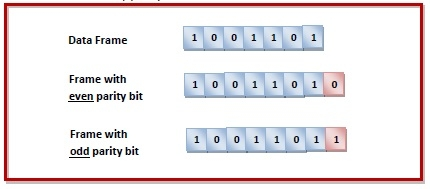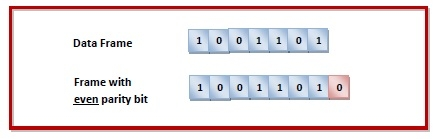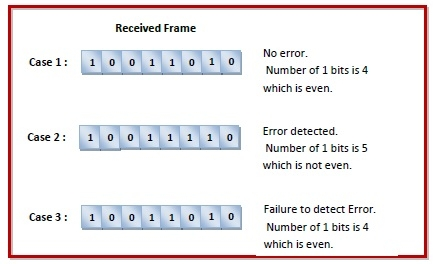# What is a Parity Bit?

A parity bit is a check bit, which is added to a block of data for error detection purposes. It is used to validate the integrity of the data. The value of the parity bit is assigned either 0 or 1 that makes the number of 1s in the message block either even or odd depending upon the type of parity. Parity check is suitable for single bit error detection only.

The two types of parity checking are

• Even Parity − Here the total number of bits in the message is made even.

• Odd Parity − Here the total number of bits in the message is made odd.

## Error Detection by Adding Parity Bit

Sender’s End − While creating a frame, the sender counts the number of 1s in it and adds the parity bit in following way

• In case of even parity − If number of 1s is even, parity bit value is 0. If number of 1s is odd, parity bit value is 1.

• In case of odd parity − If number of 1s is odd, parity bit value is 0. If number of 1s is even, parity bit value is 1.Receiver’s End − On receiving a frame, the receiver counts the number of 1s in it. In case of even parity check, if the count of 1s is even, the frame is accepted, otherwise it is rejected. In case of odd parity check, if the count of 1s is odd, the frame is accepted, otherwise it is rejected.

## Example

Suppose that a sender wants to send the data 1001101 using even parity check method. It will add the parity bit as shown below.The receiver will decide whether error has occurred by counting whether the total number of 1s is even. When the above frame is received, three cases may occur namely, no error, single bit error detection and failure to detect multiple bits error. This is illustrated as follows